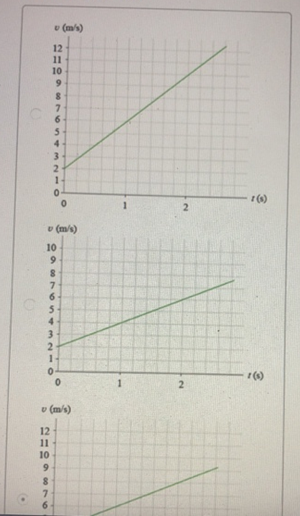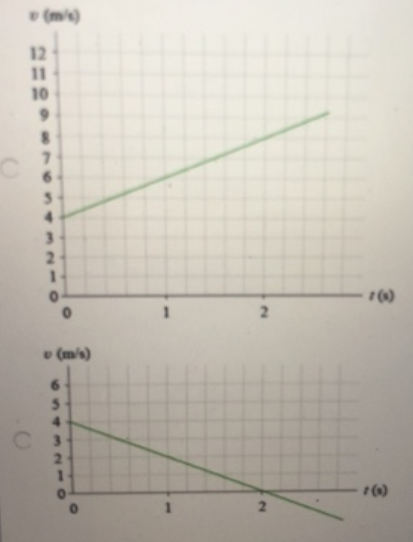# Problem: Which diagram shows the equation v = 2t + 4?

###### FREE Expert Solution
83% (30 ratings)###### Problem Details

Which diagram shows the equation v = 2t + 4?